# How to Start Using COUNTIF, SUMIF, and AVERAGEIF in Excel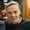Learning Microsoft Excel is all about adding more and more formulas and functions to your toolbelt. Combine enough of these, and you can do practically anything with a spreadsheet.

In this tutorial, you'll learn how to use three powerful Excel formulas: SUMIF, COUNTIF, and AVERAGEIF.

In the screenshot above, you can see that we have a list of transactions on the left side. If I want to keep an eye on my spending, I can use these three formulas to monitor it.

On the right side, the Dining Out Expense box uses the three formulas to help me track my expenses:

• COUNTIF - Used to count the number of times that "Restaurant" appears in the list.
• SUMIF - Calculates the total expense for items labeled "Restaurant."
• AVERAGIEF - Averages out all of my "Restaurant" expenses in the list.

More generically, here's what each of those formulas do for you, and how you might use them to your advantage:

• SUMIF - Add values if a condition is met, such as adding up all purchases from one category.
• COUNTIF - Count up the number of items that meet a condition, such as counting the number of times a name appears in a list.
• AVERAGEIF - Conditionally average values; for instance, you could average your grades for only exams.

These formulas allow you to add logic to your spreadsheet. Let's look at how to use each formula.

COUNTIF, SUMIF, and AVERAGEIF in Excel (Quick Video Tutorial)

Screencasts are one of the best ways to watch and learn a new skill, including getting started with these three key formulas in Microsoft Excel. Check out the video below to watch how I work in Excel. Make sure and download the free example workbook to use with this tutorial.

If you prefer to learn with written, step-by-step instructions, keep reading. I'll share tips for how to use these formulas and ideas for why they're useful.

## How to Use SUMIF in Excel

Use the tab titled SUMIF in the free example workbook for this section of the tutorial.

Think of SUMIF as a way to add values that meet a rule. We can add up a list of values that are from a certain category, or all values greater than or less than a specific amount.

Here's how the SUMIF formula works:

=SUMIF(Cells to check, what to check for, Sum of cells that meet the rules)

Let's go back to my restaurant expense example to learn the SUMIF formula. Below, I show a list of my transactions for the month.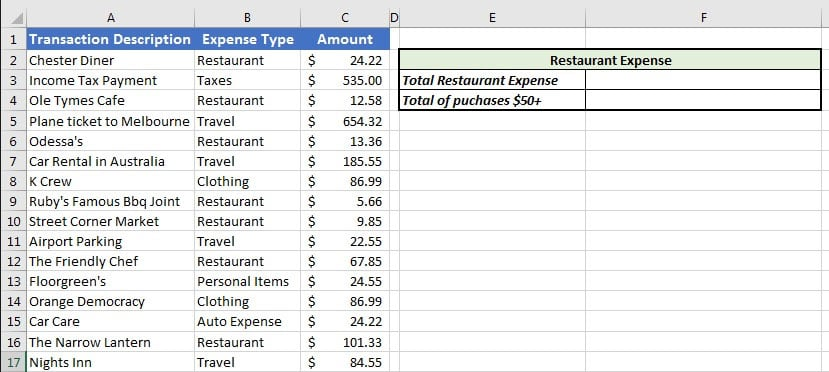I have a list of transactions, and I'm going to use SUMIF to help me keep an eye on my spending.

I want to know two things:

1. The total of what I spent at restaurants for the month.
2. All purchases greater than $50 for the month, from any category. Instead of manually adding data up, we can write two SUMIF formulas to automate the process. I'll put the results in the green Restaurant Expense box on the right side. Let's look at how. ### Total Restaurant Expense To find my total restaurant expense, I'll sum up all values with the expense type of "Restaurant", which is in column B. Here's the formula I'll use for this example: =SUMIF(B2:B17,"Restaurant",C2:C17) Notice that each section is separated by a comma. This formula does three key things: • Looks at what's in cells B2 to B17 for the category of expense • Uses "Restaurant" for the criteria of what to sum up • Uses the values in cells C2 to C17 to total up the amounts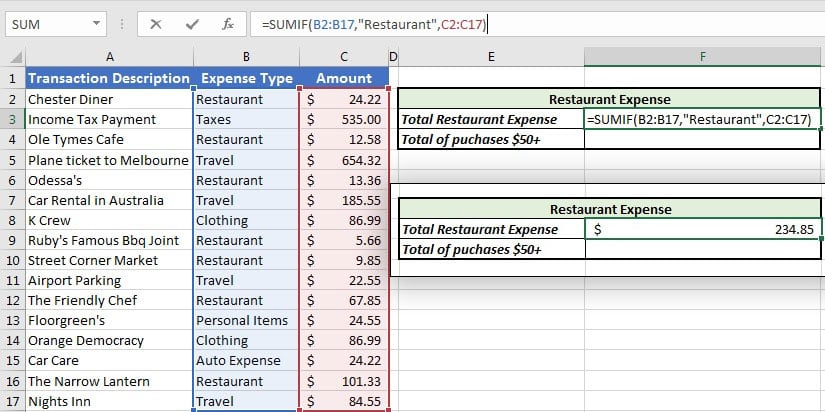In this example, I'm summing up all values from the expense type "Restaurant." When I press enter, Excel calculates the total of my restaurant expenses. Using SUMIF, it's easy to create these quick statistics to help you monitor data of certain types. ### Purchases Greater Than$50

We've checked for a specific category, but now let's sum up all values that are greater than an amount from any category. In this case, I want to find all purchases that were more than $50. Let's write a simple formula to find the sum of all purchases greater than$50:

=SUMIF(C2:C17,">50")

In this case, the formula is a bit simpler: since we're summing up the same values that we're testing (C2 to C17), we just need to specify those cells. Then, we'll add a comma and ">50" to only sum values greater than $50.Sum up all purchases greater than$50 with a simple Excel formula.

This example uses a greater than sign, but for bonus points: try to sum up all small purchases, such as all purchases $20 or less. ## How to Use COUNTIF in Excel Use the tab titled COUNTIF in the free example workbook for this section of the tutorial. While SUMIF is used to add values that meet a certain condition, COUNTIF will count up the number of times something appears in a given set of data. Here's the general format for the COUNTIF formula: =COUNTIF(cells to count, criteria to count) Using the same set of data, let's count two key pieces of information: • The number of clothing purchases I made in a month • The number of purchases$100 or greater

### Number of Clothing Purchases

My first COUNTIF will look at the expense type and count up the number of "Clothing" purchases in my transactions.

The final formula will be:

=COUNTIF(B2:B17,"Clothing")

That formula looks at the "Expense Type" column, counts up the number of times it sees clothing, and counts them. The result is 2.

### Number of $100+ Purchases Now, let's count the number of transactions that were$100 or greater in my list.

Here's the formula I'll use:

=COUNTIF(C2:C17,">100")

This is a simple, two part formula: simply point Excel to the list of data to count, and the rule to count. In this case, we're checking cells C2 to C17, for all values greater than $100.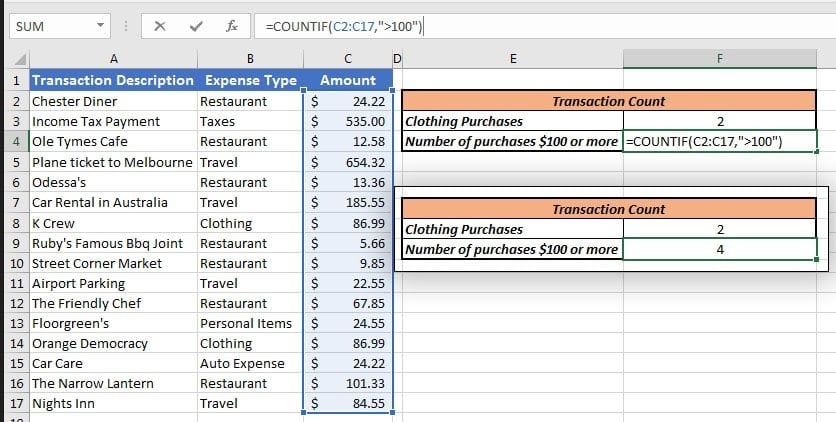Count the number of transactions$100+ with this Excel COUNTIF formula.

## How to Use AVERAGEIF in Excel

Use the tab titled AVERAGEIF in the free example workbook for this section of the tutorial.

Last up, let's look at how to use an AVERAGEIF formula. By now, it should be no surprise that AVERAGEIF can be used to average specific values, based on a condition that we'll give Excel.

The format for an AVERAGEIF formula is:

=AVERAGEIF(Cells to check, what to check for, Average of cells that meet the rules)

The format of the AVERAGEIF formula is most similar to the SUMIF formula.

Let's use the AVERAGEIF formula to calculate two key stats about my spending:

1. The average of my restaurant expenses.
2. The average of all expenses less than $25. ### Average of Restaurant Purchases To average my restaurant expenses, I'll write an AVERAGEIF formula to average all amounts based on the category. =AVERAGEIF(B2:B17,"Restaurant",C2:C17) There are three parts to this formula, each separated by a comma: • B2:B17 specifies the cells to check a condition for. Since the expense type is specified in this column. • "Restaurant" gives the formula something to look for. • Finally, C2:C17 are the cells to average out in our calculation.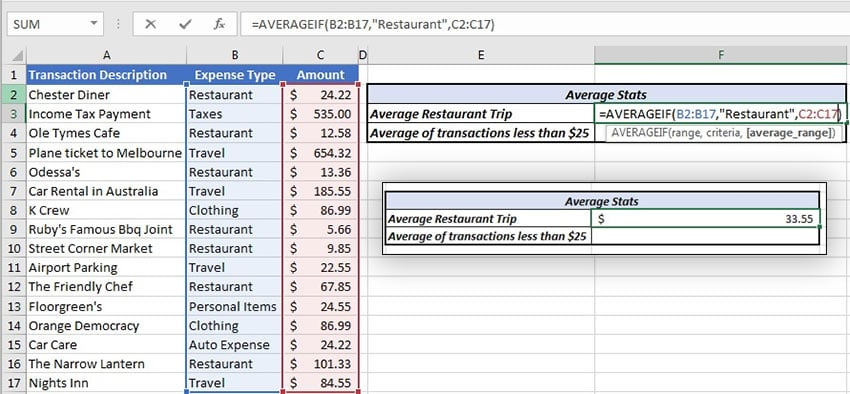Here I'm using an AVERAGEIF to average the expense of my annual restaurant trip. At the end, Excel averages out the expense of my restaurant trips. I've given it that formula. You can also try out this formula by replacing "Restaurant" with another category, like "Clothing." ### Average of Expenses Less than$25

If I'm keeping an eye on my smaller purchases and want to know my average, I can write an AVERAGEIF for all purchases less than an amount.

Here's the formula that I'll use to do that:

=AVERAGEIF(C2:C17,"<25")

This simple formula just checks the values in column C, and averages all values greater than $25.Simple Excel AVERAGEIF formula for expenses Less than$25.

## Recap and Keep Learning

In this tutorial, you learned how to use three conditional math formulas to review your data. Whether you're summing, counting, or averaging data, these functions are advanced Excel skills you can put to good use.

For all of the "IF" formulas in this tutorial, the key takeaway is that you can apply conditions to your calculations in Excel.

Learning begets learning. Here are three more Excel tutorials to keep growing:

Can you think of other ways to use these formulas to add logic and conditions to your spreadsheet? Let me know with a comment below.